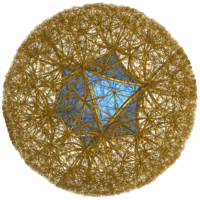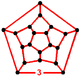# Icosahedral honeycomb

﻿
Icosahedral honeycomb
Icosahedral honeycombPoincaré disk model
Type regular hyperbolic honeycomb
Schläfli symbol {3,5,3}
Coxeter-Dynkin diagramCells icosahedron {3,5}
Faces triangle {3}
Edge figure triangle {3}
Vertex figuredodecahedron
Cells/edge {3,5}3
Cells/vertex {3,5}12
Dual Self-dual
Coxeter group J3, [3,5,3]
Properties Regular

The icosahedral honeycomb is one of four regular space-filling tessellations (or honeycombs) in hyperbolic 3-space.

Three icosahedra surround each edge, and 12 icosahedra surround each vertex, in a regular dodecahedral vertex figure.

The dihedral angle of a Euclidean icosahedron is 138.2°, so it is impossible to fit three icosahedra around an edge in Euclidean 3-space. However in hyperbolic space, properly scaled icosahedra can have dihedral angles exactly 120 degrees, so three of these fit around an edge.

## Related honeycombs

There are nine uniform honeycombs in the [3,5,3] Coxeter group family, including this regular form as well as the bitruncated form, t1,2{3,5,3},, also called truncated dodecahedral honeycomb, each of whose cells is a truncated dodecahedron.